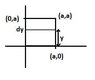# Calculating Flux through Square

• Faint

## Homework Statement

The electric field through a region of space is given by:

E = (-1.10×10^4 N/Cm2)xyi + (-6.20×10^4 N/Cm)xj + (-6.60×10^4 N/Cm)yk

A square is located in the x-y plane, at z = 0, as shown in the figure below. The sides of the square have length 7.86 cm. What is the electric flux through the square?

Flux = E * dA

## The Attempt at a Solution

The square is in the x-y plane, so we can ignore i and j components of the electric field. The thing that is throwing me off is the y in the k component of the electric field. This is what I tried:

$$\int^{0}_{0.786}(-6.6*10^4)*y^2*y$$

But it gives me an incorrect answer. Help?

The square is in the x-y plane, so you can not ignore i j component
you can just put z=0 in eqn of E ...(although z is not in us in that)

The square is in the x-y plane, so you can not ignore i j component
you can just put z=0 in eqn of E ...(although z is not in us in that)

But aren't the i and j components not passing through the square, thus affecting the answer?

But aren't the i and j components not passing through the square, thus affecting the answer?

I can't understand you.

Flux can be defined as "the rate of flow of energy or particles across a given surface." The i and j components would be parallel to the x-y plane, not perpendicular, so it's not crossing the plane, thus no flux. Does this make more sense/is this correct?

Yes you're right!

I don't know what i was thinking! Need more sleep :zzz:

sorry for that

Yes you only need to consider the k component

Yes you're right!

I don't know what i was thinking! Need more sleep :zzz:

sorry for that

Yes you only need to consider the k component

Do I need to take the integral like I did then? How does having the y variable in the k component affect the answer?

You need to do a double integral

∫∫ Ez dy dx

assuming that the square is in only the first quadrant and has one point at the origin
both integrals bounded from 0 to .0786 m
=∫∫ ((-6.60×10^4 N/Cm)y) dy dx
=-6.60×10^4 N/Cm ∫(y^2)/2 dx
=(-6.60×10^4 N/Cm)/2*(.0786m)^2 *∫dx
=(-6.60×10^4 N/Cm)/2*(.0786m)^2 *.0786m
=(-6.60×10^4 N/Cm)/2*(.0786m)^3
=-16.0245 V*m

Last edited:
You need to do a double integral

∫∫ Ez dy dx

assuming that the square is in only the first quadrant and has one point at the origin
both integrals bounded from 0 to .0786 m
=∫∫ ((-6.60×10^4 N/Cm)y) dy dx
=-6.60×10^4 N/Cm ∫(y^2)/2 dx
=(-6.60×10^4 N/Cm)/2*(.0786m)^2 *∫dx
=(-6.60×10^4 N/Cm)/2*(.0786m)^2 *.0786m
=(-6.60×10^4 N/Cm)/2*(.0786m)^3
=-16.0245 V*m

That worked! Thanks!

You need not to double integrate!
It can be solved with much simpler calculus

consider the square to start at (0,0) and of sides a <see pic>
take an element of width dy at distance y from X axis
its area is a(dy)
so d(flux) = ∫E(ds) = ∫(-6.6*10^4)y (a dy)
solve it for y ranging from 0 to a

and you have the same answer -16.0245

#### Attachments

•Q.jpg
3.6 KB · Views: 631
You need not to double integrate!
It can be solved with much simpler calculus
Yes in this case you are right... but what if the z component of the E field were 3xy.. the simpler method would no longer work. In this case you would have to double integrate. Double integrals work in a more general setting.

Ahh yes and Faint... you may not have seen double integrals or triple integrals before but they are not extremely difficult. For each integration you just pretend that all variables except the one you are integrating are constants. A very nice explanation of multiple integrations can be found here on Khan Academy video lectures: